Definitions of Square Dance Calls and Concepts

\$B%+%C%W%k\$^\$?\$O(B mini wave \$B\$+\$i!%(B

Hinge \$B\$r\$7\$F(B, \$B;X<(\$5\$l\$??M\$,(B flow \$B\$NJ}\$X(B U-Turn Back (Roll twice) \$B\$r\$7\$^\$9!%(B

\$B%+%C%W%k\$^\$?\$O(B mini wave \$B\$G=*\$o\$j\$^\$9!%\$3\$l\$O(B 2 \$B%Q!<%H\$N%3!<%k\$G\$9!%(B

\$BCm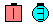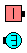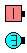Patch The Belles\$B\$NA0(B Hinge\$B\$N8e(B original Belles Roll twice \$B\$N8e(B (\$B=*\$o\$j(B)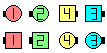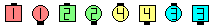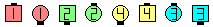Patch The Centers\$B\$NA0(B Hinge\$B\$N8e(B original Centers Roll twice \$B\$N8e(B (\$B=*\$o\$j(B)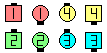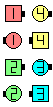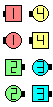Patch The Leaders\$B\$NA0(B Hinge\$B\$N8e(B original Leaders Roll twice \$B\$N8e(B (\$B=*\$o\$j(B)

anything Like A Patch The anyone [C3AV]: anything call \$B\$r\$7\$F(B, \$B;X<(\$5\$l\$??M\$,(B U-Turn Back \$B\$r\$7\$^\$9!%\$3\$N>l9g%3!<%i!<\$O(B, \$B;X<(\$9\$k?M\$K\$O[#Kf\$5\$,\$J\$\$\$h\$&\$K(B (\$BNc\$(\$P(B, Girls, Head Men, ...) \$B\$7\$J\$1\$l\$P\$J\$j\$^\$;\$s!#\$=\$&\$G\$J\$\$\$H(B, \$B%@%s%5!<\$O\$@\$l\$,(B U-Turn Back \$B\$9\$k\$N\$+J,\$+\$i\$J\$/\$J\$j\$^\$9!%Nc\$H\$7\$F(B, In Roll Circulate Like A Patch The Centers \$B\$G\$O(B, \$B%*%j%8%J%k\$N(B centers \$B\$J\$N\$+7k2L\$N(B centers \$B\$J\$N\$+J,\$+\$j\$^\$;\$s!%(B